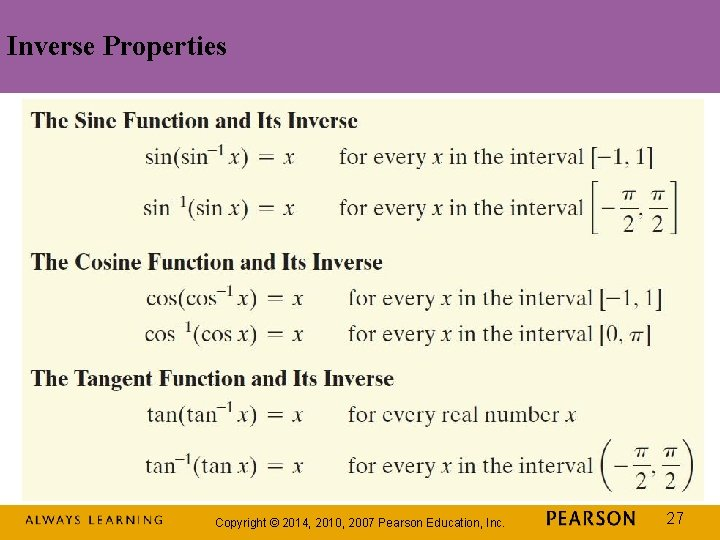Chapter 4 Trigonometric Functions 4 7 Inverse Trigonometric

• Slides: 28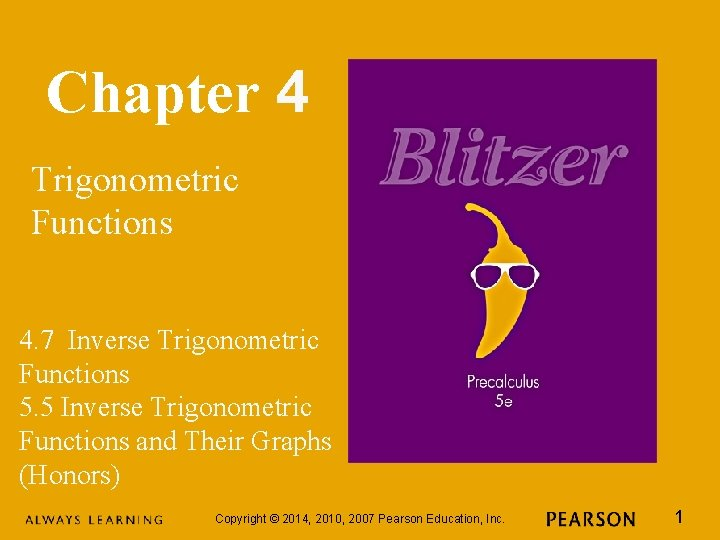Chapter 4 Trigonometric Functions 4. 7 Inverse Trigonometric Functions 5. 5 Inverse Trigonometric Functions and Their Graphs (Honors) Copyright © 2014, 2010, 2007 Pearson Education, Inc. 1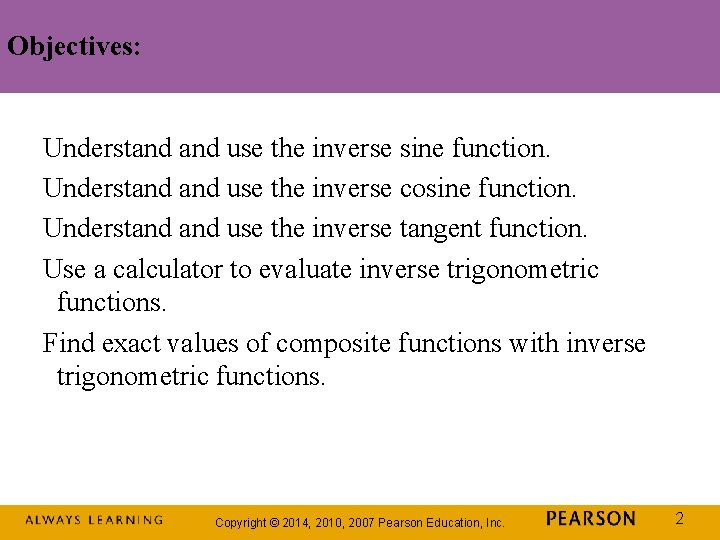Objectives: Understand use the inverse sine function. Understand use the inverse cosine function. Understand use the inverse tangent function. Use a calculator to evaluate inverse trigonometric functions. Find exact values of composite functions with inverse trigonometric functions. Copyright © 2014, 2010, 2007 Pearson Education, Inc. 2Inverse Functions Here are some helpful things to remember from our earlier discussion of inverse functions: If no horizontal line intersects the graph of a function more than once, the function is one-to-one and has an inverse function. If the point (a, b) is on the graph of f, then the point (b, a) is on the graph of the inverse function, denoted f – 1. The graph of f – 1 is a reflection of the graph of f about the line y = x. Copyright © 2014, 2010, 2007 Pearson Education, Inc. 3The Inverse Sine Function The horizontal line test shows that the sine function is not one-to-one; y = sin x has an inverse function on the restricted domain Copyright © 2014, 2010, 2007 Pearson Education, Inc. 4The Inverse Sine Function (continued) Copyright © 2014, 2010, 2007 Pearson Education, Inc. 5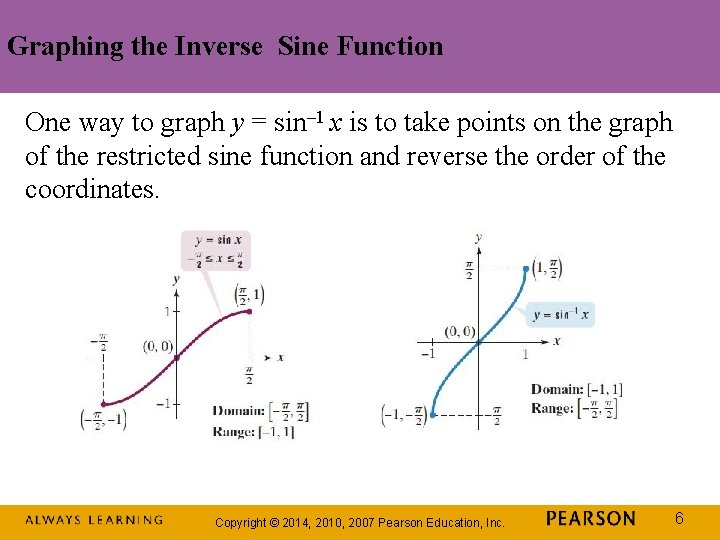Graphing the Inverse Sine Function One way to graph y = sin– 1 x is to take points on the graph of the restricted sine function and reverse the order of the coordinates. Copyright © 2014, 2010, 2007 Pearson Education, Inc. 6Graphing the Inverse Sine Function (continued) Another way to obtain the graph of y = sin– 1 x is to reflect the graph of the restricted sine function about the line y = x. Copyright © 2014, 2010, 2007 Pearson Education, Inc. 7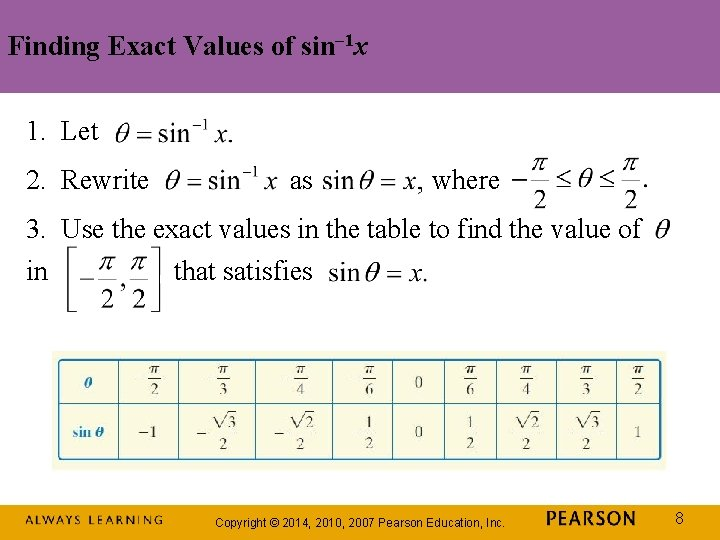Finding Exact Values of sin– 1 x 1. Let 2. Rewrite as where 3. Use the exact values in the table to find the value of in that satisfies Copyright © 2014, 2010, 2007 Pearson Education, Inc. 8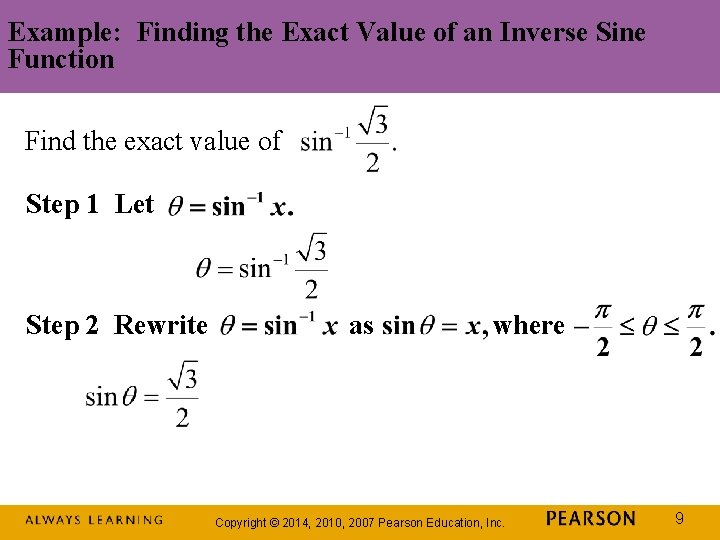Example: Finding the Exact Value of an Inverse Sine Function Find the exact value of Step 1 Let Step 2 Rewrite as where Copyright © 2014, 2010, 2007 Pearson Education, Inc. 9Example: Finding the Exact Value of an Inverse Sine Function (continued) Find the exact value of Step 3 Use the exact value in the table to find the value of in The angle in that satisfies whose sine is is Copyright © 2014, 2010, 2007 Pearson Education, Inc. 10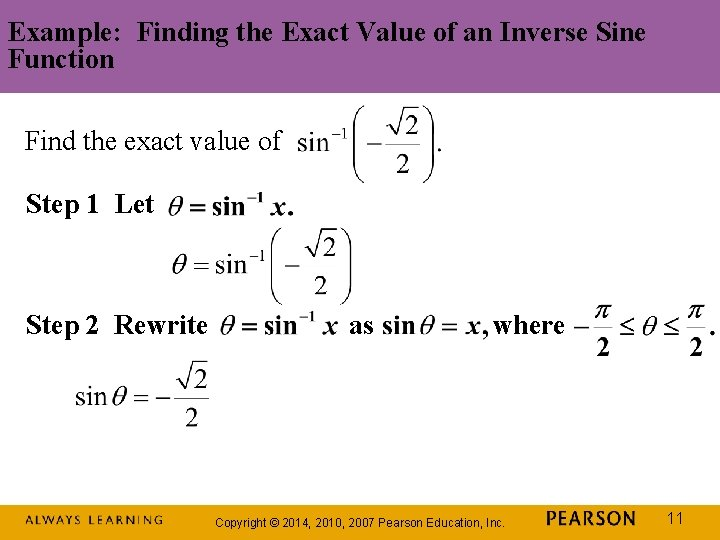Example: Finding the Exact Value of an Inverse Sine Function Find the exact value of Step 1 Let Step 2 Rewrite as where Copyright © 2014, 2010, 2007 Pearson Education, Inc. 11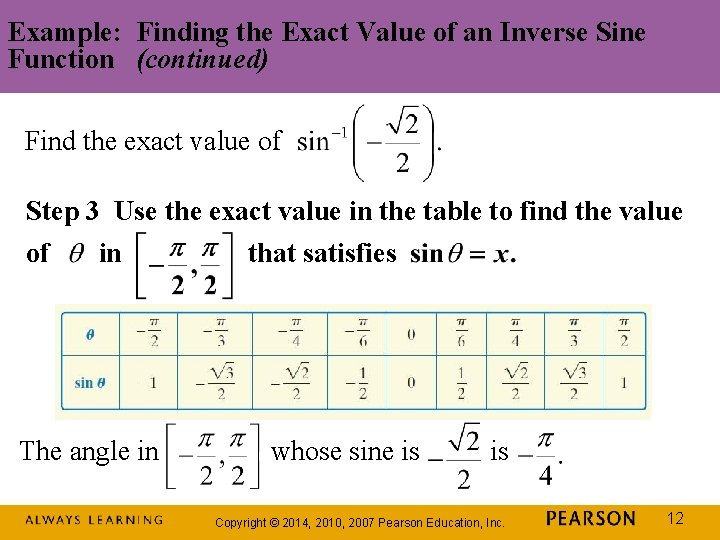Example: Finding the Exact Value of an Inverse Sine Function (continued) Find the exact value of Step 3 Use the exact value in the table to find the value of in The angle in that satisfies whose sine is is Copyright © 2014, 2010, 2007 Pearson Education, Inc. 12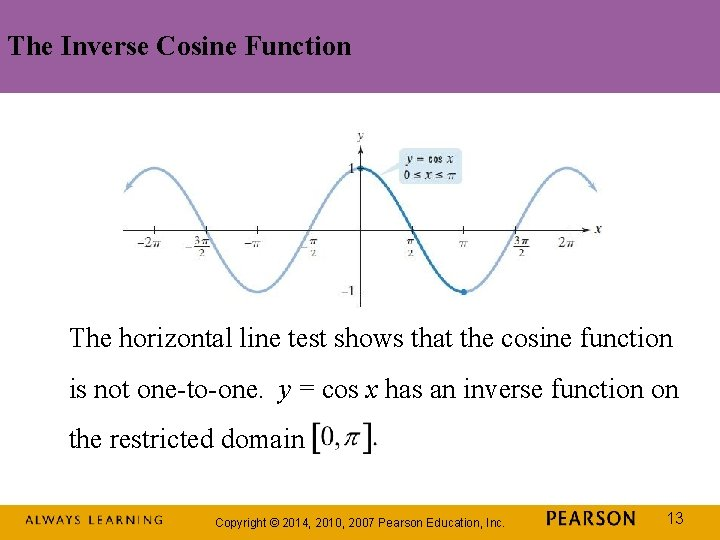The Inverse Cosine Function The horizontal line test shows that the cosine function is not one-to-one. y = cos x has an inverse function on the restricted domain Copyright © 2014, 2010, 2007 Pearson Education, Inc. 13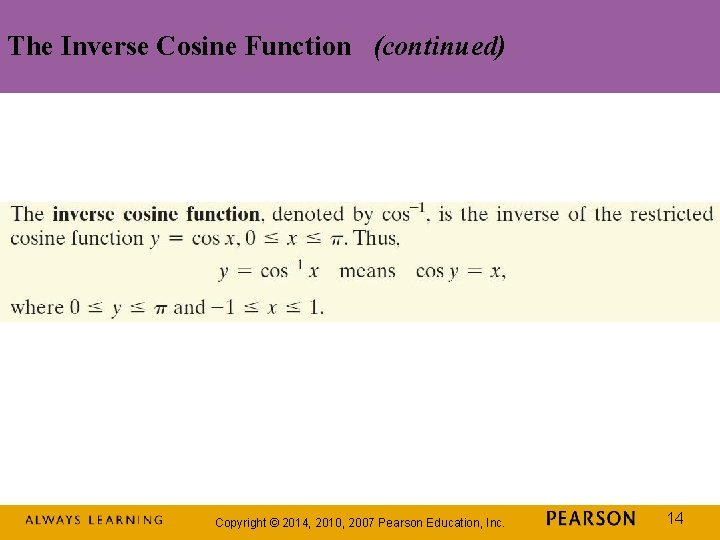The Inverse Cosine Function (continued) Copyright © 2014, 2010, 2007 Pearson Education, Inc. 14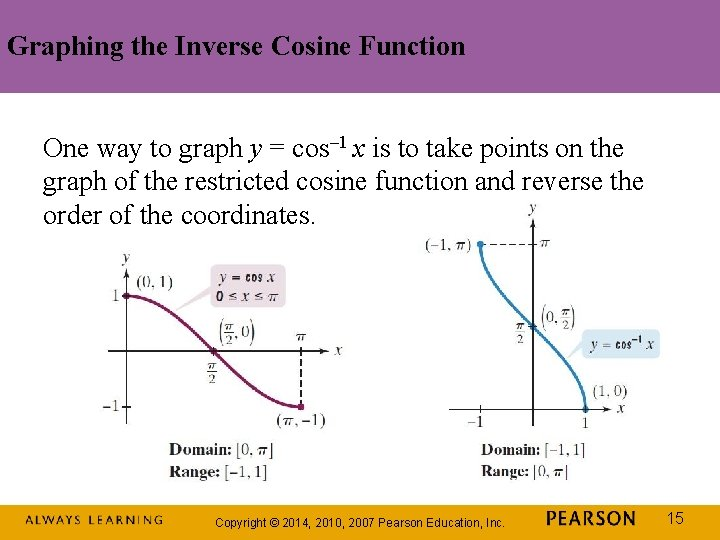Graphing the Inverse Cosine Function One way to graph y = cos– 1 x is to take points on the graph of the restricted cosine function and reverse the order of the coordinates. Copyright © 2014, 2010, 2007 Pearson Education, Inc. 15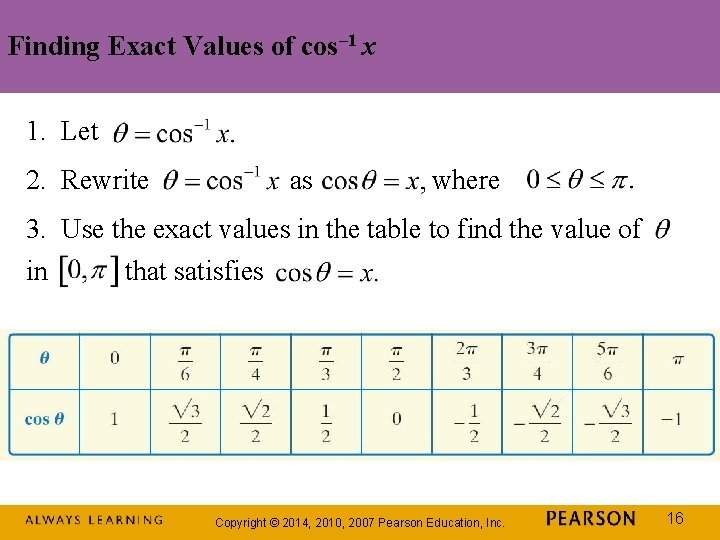Finding Exact Values of cos– 1 x 1. Let 2. Rewrite as where 3. Use the exact values in the table to find the value of in that satisfies Copyright © 2014, 2010, 2007 Pearson Education, Inc. 16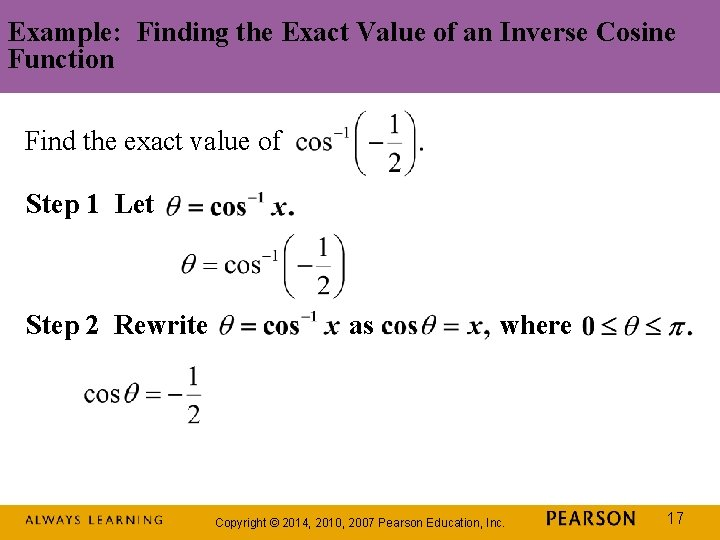Example: Finding the Exact Value of an Inverse Cosine Function Find the exact value of Step 1 Let Step 2 Rewrite as where Copyright © 2014, 2010, 2007 Pearson Education, Inc. 17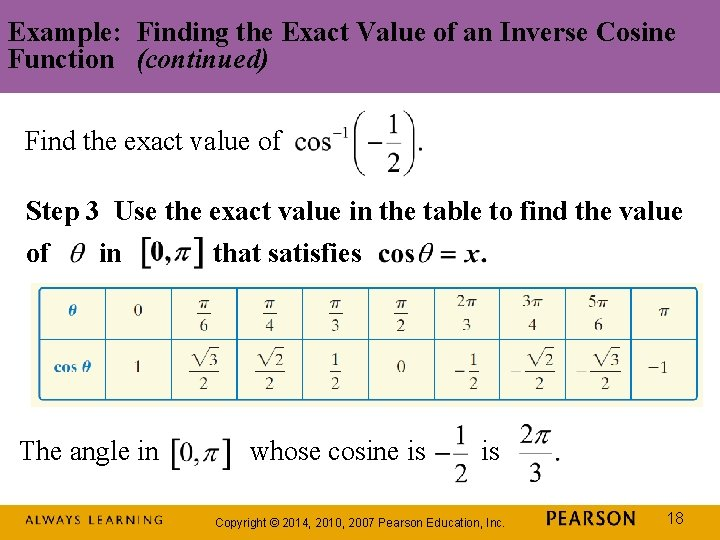Example: Finding the Exact Value of an Inverse Cosine Function (continued) Find the exact value of Step 3 Use the exact value in the table to find the value of in The angle in that satisfies whose cosine is is Copyright © 2014, 2010, 2007 Pearson Education, Inc. 18The Inverse Tangent Function The horizontal line test shows that the tangent function is not one-to-one. y = tan x has an inverse function on the restricted domain Copyright © 2014, 2010, 2007 Pearson Education, Inc. 19The Inverse Tangent Function (continued) Copyright © 2014, 2010, 2007 Pearson Education, Inc. 20Graphing the Inverse Tangent Function One way to graph y = tan– 1 x is to take points on the graph of the restricted tangent function and reverse the order of the coordinates. Copyright © 2014, 2010, 2007 Pearson Education, Inc. 21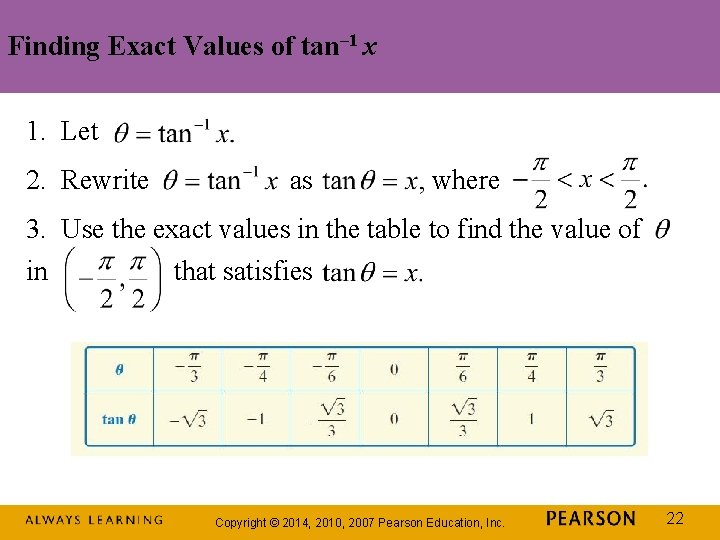Finding Exact Values of tan– 1 x 1. Let 2. Rewrite as where 3. Use the exact values in the table to find the value of in that satisfies Copyright © 2014, 2010, 2007 Pearson Education, Inc. 22Example: Finding the Exact Value of an Inverse Tangent Function Find the exact value of Step 1 Let Step 2 Rewrite as where Copyright © 2014, 2010, 2007 Pearson Education, Inc. 23Example: Finding the Exact Value of an Inverse Sine Function (continued) Find the exact value of Step 3 Use the exact value in the table to find the value of in The angle in that satisfies whose tangent is – 1 is Copyright © 2014, 2010, 2007 Pearson Education, Inc. 24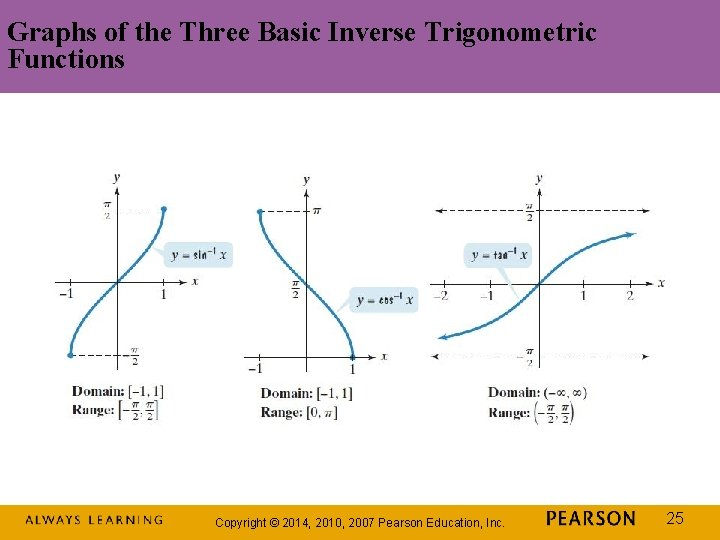Graphs of the Three Basic Inverse Trigonometric Functions Copyright © 2014, 2010, 2007 Pearson Education, Inc. 25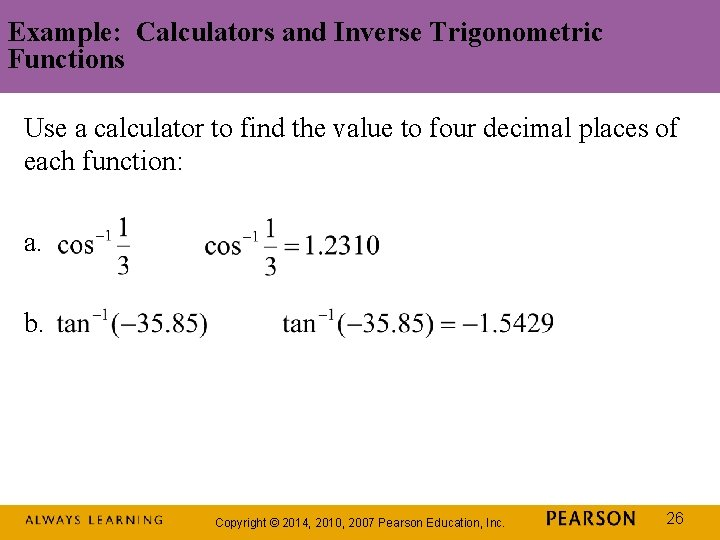Example: Calculators and Inverse Trigonometric Functions Use a calculator to find the value to four decimal places of each function: a. b. Copyright © 2014, 2010, 2007 Pearson Education, Inc. 26